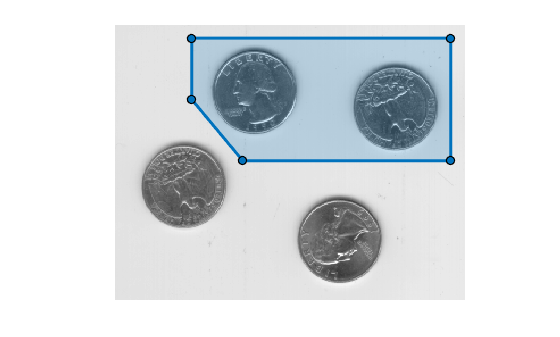# regionfill

Fill specified regions in image using inward interpolation

## Syntax

``J = regionfill(I,mask)``
``J = regionfill(I,x,y)``

## Description

example

````J = regionfill(I,mask)` fills the regions in image `I` specified by `mask`. Nonzero pixels in `mask` designate the pixels of image `I` to fill. You can use `regionfill` to remove objects in an image or to replace invalid pixel values using their neighbors.```

example

````J = regionfill(I,x,y)` fills the region in image `I` corresponding to the polygon with vertices specified by `x` and `y`. ```

## Examples

collapse all

Read and display a grayscale image.

```I = imread('eight.tif'); imshow(I)```Specify the x- and y-coordinates of a polygon that completely surrounds one of the coins in the image.

```x = [222 272 300 270 221 194]; y = [21 21 75 121 121 75];```

Fill the polygon by using the `regionfill` function.

`J = regionfill(I,x,y);`

Display the filled image.

```imshow(J) title('Filled Image with One Fewer Coin')```Read and display a grayscale image.

```I = imread('eight.tif'); imshow(I)```Specify the vertices of a polygon ROI that completely surrounds two of the coins by using the `drawpolygon` function. Specify the 'Position' name-value pair argument as the x-coordinates and y-coordinates of the polygon vertices. If you want to draw the polygon interactively, then omit the 'Position' name-value pair argument.

```x = [68 296 296 113 68]; y = [12 12 120 120 66]; roi = drawpolygon(gca,'Position',[x;y]');```Create a mask image in which the ROI is `true` and the background is `false`. Display the mask.

```mask = createMask(roi); imshow(mask)```Fill the regions in the input image using the mask image. Display the filled image.

```J = regionfill(I,mask); imshow(J)```## Input Arguments

collapse all

Grayscale image, specified as a 2-D numeric matrix of size greater than or equal to 3-by-3.

Data Types: `single` | `double` | `int8` | `int16` | `int32` | `uint8` | `uint16` | `uint32`

Mask image, specified as a logical matrix or numeric matrix of the same size as `I`. For numeric input, any nonzero pixels are considered to be `1` (`true`).

Data Types: `single` | `double` | `int8` | `int16` | `int32` | `int64` | `uint8` | `uint16` | `uint32` | `uint64` | `logical`

x-coordinates of polygon vertices, specified as a numeric vector. `x` must be the same length as `y`.

Example: `[222 272 300 270 221 194];`

Data Types: `single` | `double` | `int8` | `int16` | `int32` | `uint8` | `uint16` | `uint32`

y-coordinates of polygon vertices, specified as a numeric vector. `y` must be the same length as `x`.

Example: `[21 21 75 121 121 75];`

Data Types: `single` | `double` | `int8` | `int16` | `int32` | `uint8` | `uint16` | `uint32`

## Output Arguments

collapse all

Filled grayscale image, returned as a 2-D numeric array. `J` has the same size and class as `I`.

## Algorithms

`regionfill` smoothly interpolates inward from the pixel values on the outer boundary of the regions. `regionfill` calculates the discrete Laplacian over the regions and solves the Dirichlet boundary value problem.

## Version History

Introduced in R2015a

expand all